# ​ Does a reaction occur when aqueous solutions of lead(II) acetate and aluminum sulfate are combined?

Does a reaction occur when aqueous solutions of lead(II) acetate and aluminum sulfate are combined?

O yes O no

If a reaction does occur, write the net ionic equation.

Use the solubility rules provided in the OWL Preparation Page to determine the solubility of compounds

Use the pull-down boxes to specify states such as (aq) or (e).

If a box is not leave it blank. 9 more group attempts remaining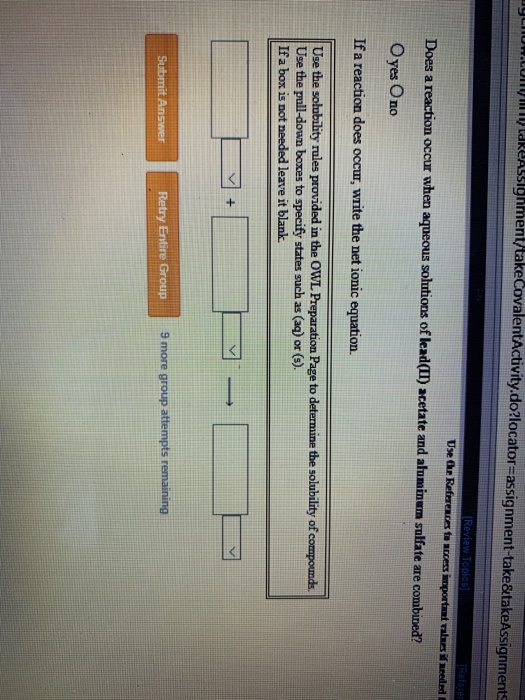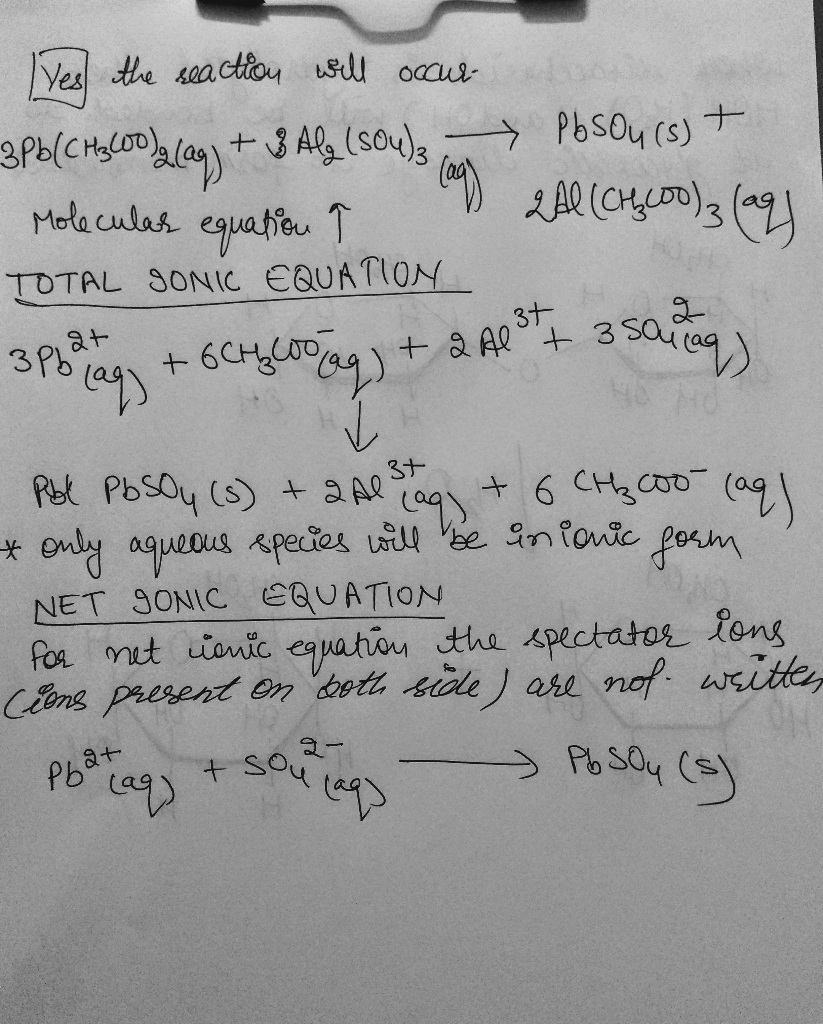#### Earn Coin

Coins can be redeemed for fabulous gifts.

Similar Homework Help Questions
• ### Does a reaction occur when aqueous solutions of lead(II) acetate and zinc bromide are combined? O...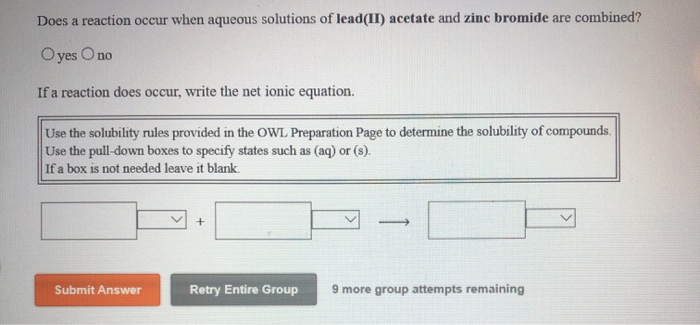Does a reaction occur when aqueous solutions of lead(II) acetate and zinc bromide are combined? O yes no If a reaction does occur, write the net ionic equation. Use the solubility rules provided in the OWL Preparation Page to determine the solubility of compounds, Use the pull-down boxes to specify states such as (aq) or (8). If a box is not needed leave it blank. Submit Answer Retry Entire Group 9 more group attempts remaining

• ### Does a reaction occur when aqueous solutions of manganese(II) sulfate and calcium nitrate are combined? yesO...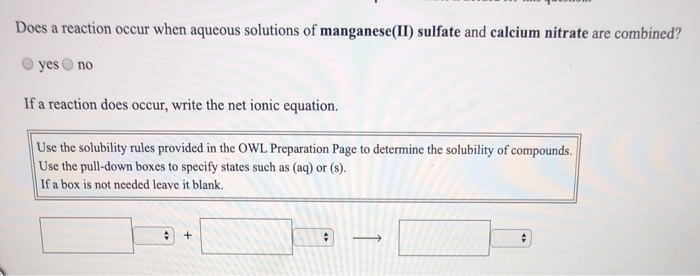Does a reaction occur when aqueous solutions of manganese(II) sulfate and calcium nitrate are combined? yesO no If a reaction does occur, write the net ionic equation. Use the solubility rules provided in the OWL Preparation Page to determine the solubility of compounds Use the pull-down boxes to specify states such as (aq) or (s). If a box is not needed leave it blank. Does a reaction occur when aqueous solutions of sodium nitrate and ammonium carbonate are combined? yes...

• ### Does a reaction occur when aqueous solutions of iron(II) nitrate and copper(II) sulfate are combined? O...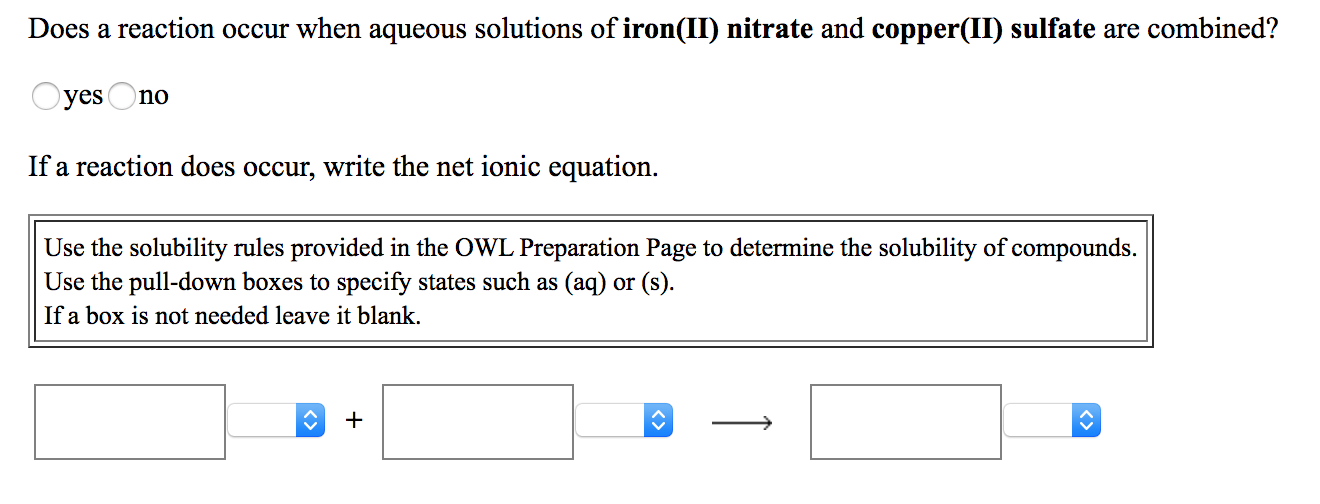Does a reaction occur when aqueous solutions of iron(II) nitrate and copper(II) sulfate are combined? O yes no If a reaction does occur, write the net ionic equation. Use the solubility rules provided in the OWL Preparation Page to determine the solubility of compounds. Use the pull-down boxes to specify states such as (aq) or (s). If a box is not needed leave it blank. Does a reaction occur when aqueous solutions of silver(I) nitrate and manganese(II) sulfate are combined?...

• ### Does a reaction occur when aqueous solutions of sodium carbonate and lead(II) acetate are combined? yes no If a rea...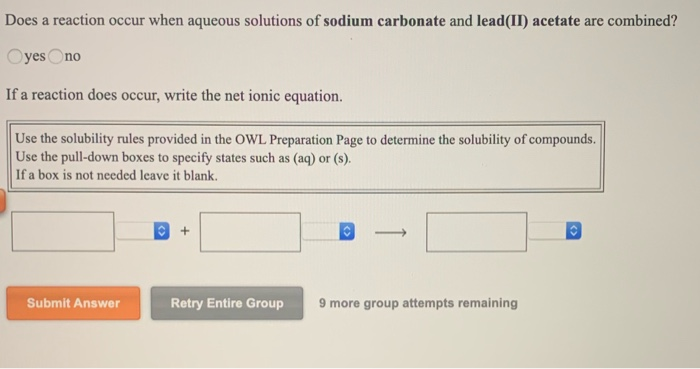Does a reaction occur when aqueous solutions of sodium carbonate and lead(II) acetate are combined? yes no If a reaction does occur, write the net ionic equation. Use the solubility rules provided in the OWL Preparation Page to determine the solubility of compounds. Use the pull-down boxes to specify states such as (aq) or (s). If a box is not needed leave it blank. Submit Answer Retry Entire Group 9 more group attempts remaining

• ### Does a reaction occur when aqueous solutions of sodium iodide and potassium carbonate are combined? O...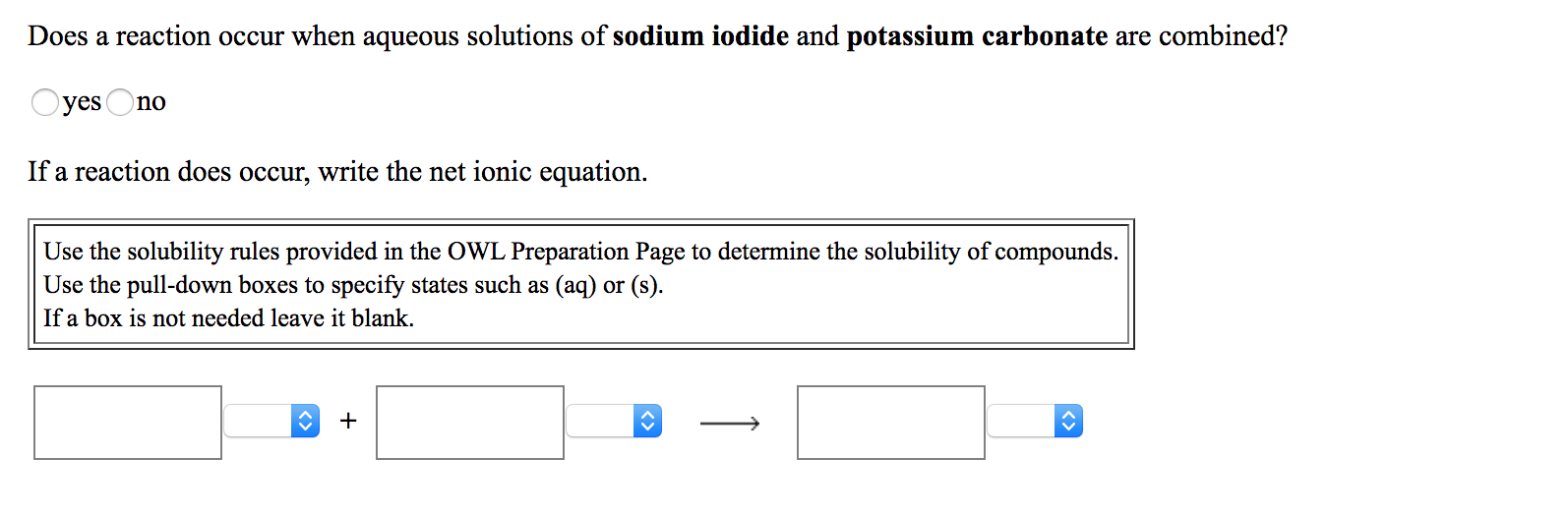Does a reaction occur when aqueous solutions of sodium iodide and potassium carbonate are combined? O yes no If a reaction does occur, write the net ionic equation. Use the solubility rules provided in the OWL Preparation Page to determine the solubility of compounds. Use the pull-down boxes to specify states such as (aq) or (s). If a box is not needed leave it blank. Does a reaction occur when aqueous solutions of aluminum acetate and ammonium carbonate are combined?...

• ### Does a reaction occur when aqueous solutions of sodium nitrate and potassium sulfide are combined? yes...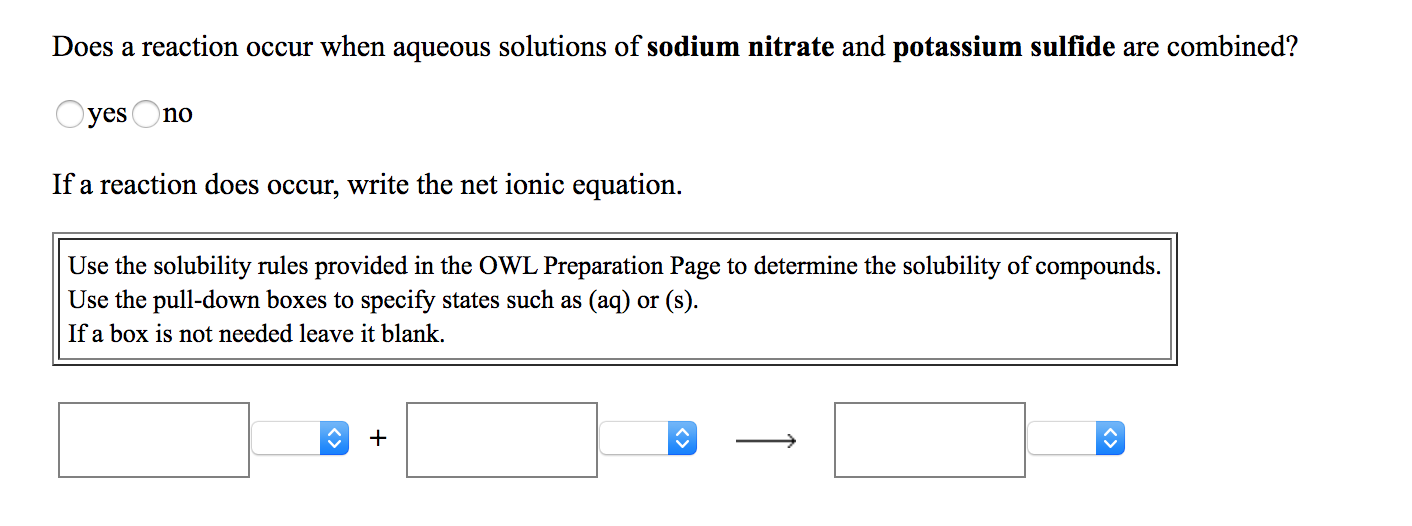Does a reaction occur when aqueous solutions of sodium nitrate and potassium sulfide are combined? yes no If a reaction does occur, write the net ionic equation. Use the solubility rules provided in the OWL Preparation Page to determine the solubility of compounds. Use the pull-down boxes to specify states such as (aq) or (s). If a box is not needed leave it blank. Does a reaction occur when aqueous solutions of lead(II) nitrate and ammonium sulfide are combined? O...

• ### Does a reaction occur when aqueous solutions of sodium carbonate and barium hydroxide are combined? no...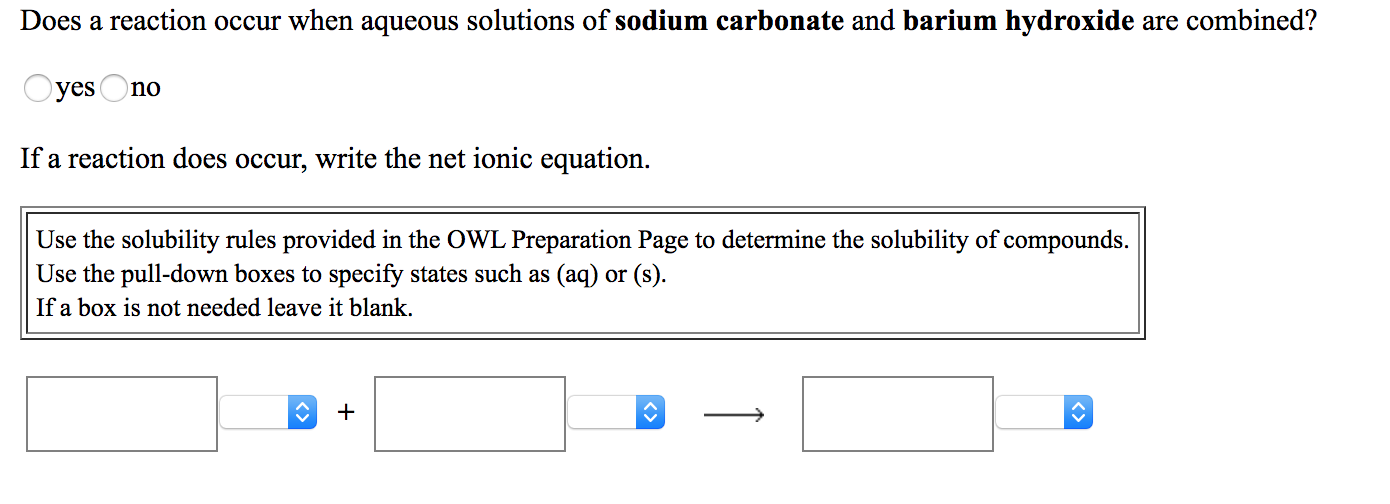Does a reaction occur when aqueous solutions of sodium carbonate and barium hydroxide are combined? no yes If a reaction does occur, write the net ionic equation Use the solubility rules provided in the OWL Preparation Page to determine the solubility of compounds. Use the pull-down boxes to specify states such as (aq) or (s) If a box is not needed leave it blank. Does a reaction occur when aqueous solutions of iron(II) nitrate and calcium bromide are combined? yes...

• ### Does a reaction occur when aqueous solutions of chromium(III) acetate and zinc bromide are combined? yes...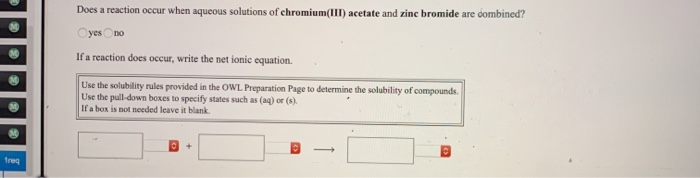Does a reaction occur when aqueous solutions of chromium(III) acetate and zinc bromide are combined? yes no If a reaction does occur, write the net ionic equation Use the solubility rules provided in the OWL Preparation Page to determine the solubility of compounds. Use the pull-down boxes to specify states such as (aq) or (s) If aber is not needed leave it blank

• ### Does a reaction occur when aqueous solutions of iron(III) bromide and silver(I) acetate are combined? yes...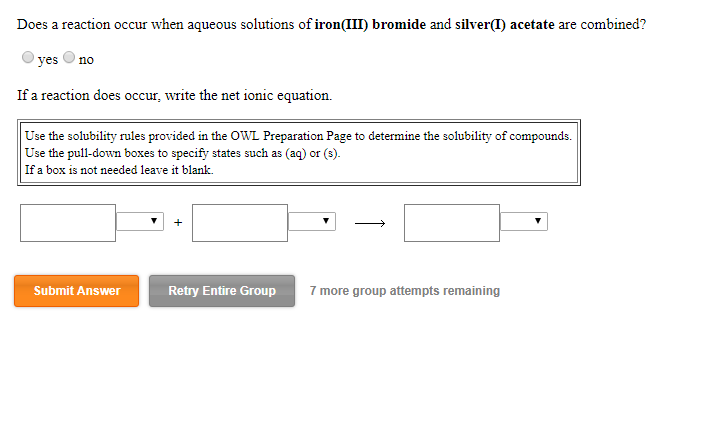Does a reaction occur when aqueous solutions of iron(III) bromide and silver(I) acetate are combined? yes no If a reaction does occur, write the net ionic equation Use the solubility rules provided in the OWL Preparation Page to determine the solubility of compounds Use the pull-down boxes to specify (aq) or (s) states such as If a box is not needed leave it blank. Submit Answer Retry Entire Group 7 more group attempts remaining

• ### 10 luCU TUI is question. Does a reaction occur when aqueous solutions of iron(II) sulfate and...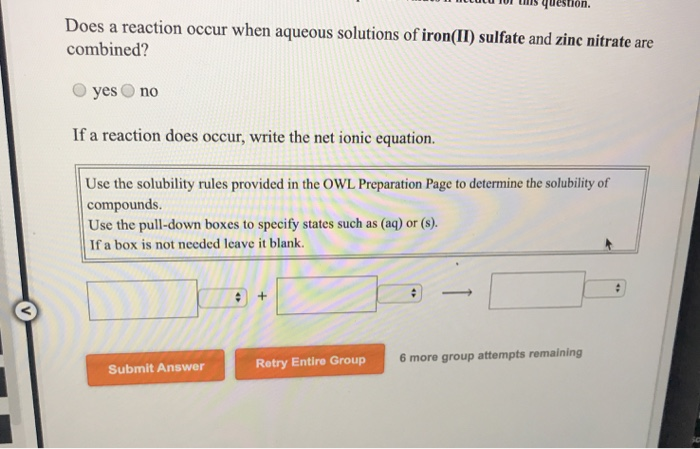10 luCU TUI is question. Does a reaction occur when aqueous solutions of iron(II) sulfate and zinc nitrate are combined? yes no If a reaction does occur, write the net ionic equation. Use the solubility rules provided in the OWL Preparation Page to determine the solubility of compounds. Use the pull-down boxes to specify states such as (aq) or (8) If a box is not needed leave it blank. 6 more group attempts remaining Submit Answer Retry Entire Group